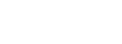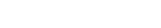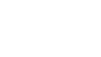## Questions1. Evaluate:-2. Find the values of a which satisfy the integral1.2. (x2 + 1) dx = 2aa3+ a – 0 =2a
3     1
a3+ 3a= 6a
a3= 3a
(a3 – 3a) = 0
a(a2 – 3) = 0
a = 0
or 3 = ± 1.732